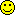LCA s.e.'s for estimates in probabili...Message/Authorbmuthen posted on Wednesday, October 16, 2002 - 5:59 am
Here is how Mplus gets the LCA s.e.'s for the binary item parameters conditional on class in the probability scale.

After the ML solution in the usual logit scale has been obtained, the s.e.'s for the corresponding probabilities are computed using the Delta Method. This method draws on a Taylor expansion, boiling down to the following.

Note that with binary outcomes you get the probability from the logit by

(1) P = 1/(1+exp(-L)).

The variance, that is the squared s.e., is obtained by the Delta Method via
the first-order derivatives as

(2) V(P) = dP/dL * V(L)* dP/dL,

where V(L) is the square of the s.e. for the regular Mplus estimates inlogit scale.

(dP/dL)^2 = P(1-P) ,

resulting in the formula Mplus uses

s.e.(P) = P(1-P)*s.e.(L)Karoline Bading posted on Friday, March 21, 2014 - 2:20 am
hello mr. muthen,

i have a question regarding the two-tailed p-value for the estimated probabilities conditional on class membership. does the program realize it is producing a p-value for a probability (which cannot be smaller than 0) and thus calculates a one-tailed p-value?
if no, what do i have to do to get the one-tailed p-value?
divide by two?

thanksTihomir Asparouhov posted on Friday, March 21, 2014 - 10:30 am
Mplus produces 2-sided P-values. For one sided P-value divide by 2.Topics | Tree View | Search | Help/Instructions | Program Credits Administration